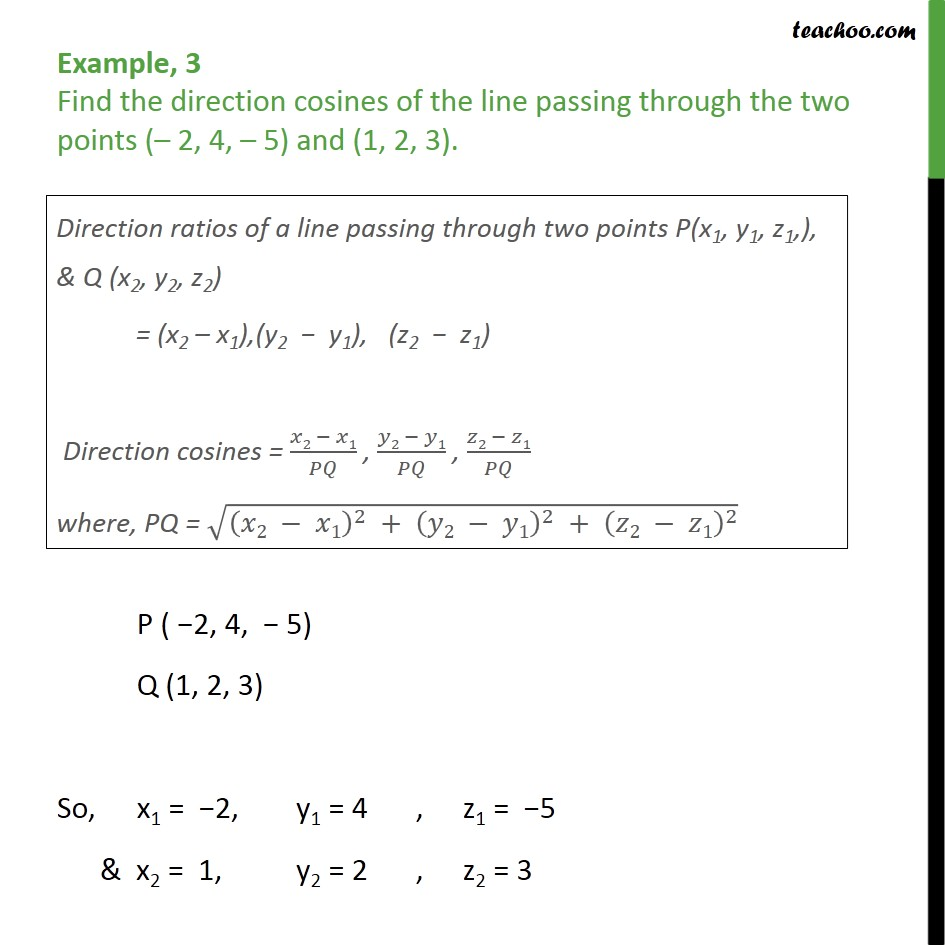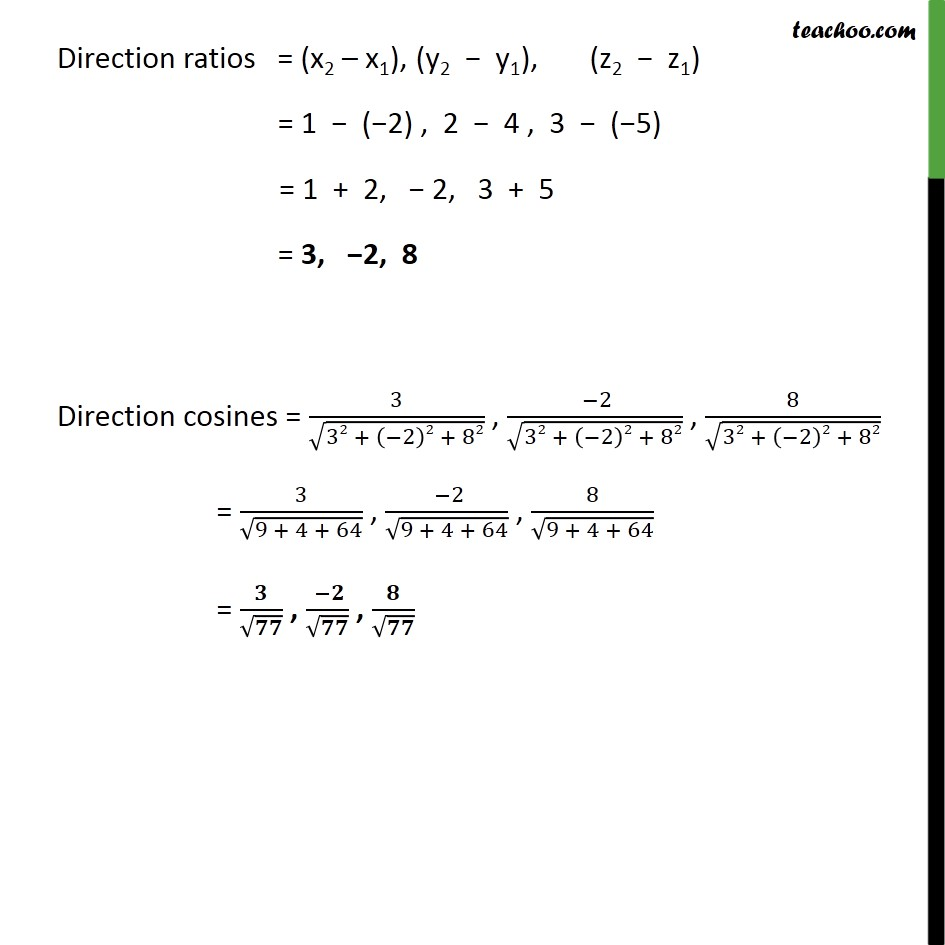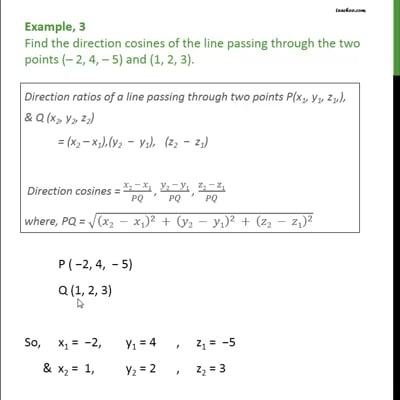Examples

Chapter 11 Class 12 Three Dimensional Geometry
Serial order wiseThis video is only available for Teachoo black users

Solve all your doubts with Teachoo Black (new monthly pack available now!)

### Transcript

Example, 3 Find the direction cosines of the line passing through the two points ( 2, 4, 5) and (1, 2, 3). P ( 2, 4, 5) Q (1, 2, 3) So, x1 = 2, y1 = 4 , z1 = 5 & x2 = 1, y2 = 2 , z2 = 3 Direction ratios = (x2 x1), (y2 y1), (z2 z1) = 1 ( 2) , 2 4 , 3 ( 5) = 1 + 2, 2, 3 + 5 = 3, 2, 8 Direction cosines = 3 32 + 2 2 + 82 , 2 32 + 2 2 + 82 , 8 32 + 2 2 + 82 = 3 9 + 4 + 64 , 2 9 + 4 + 64 , 8 9 + 4 + 64 = , ,